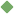modulus 1. [ noun ] (mathematics) the absolute value of a complex number Related terms: absolute_value To share this definition press "text" (Facebook, Twitter) or "link" (blog, mail) then paste text link 2. [ noun ] (mathematics) an integer that can be divided without remainder into the difference between two other integers Examples: "2 is a modulus of 5 and 9" Related terms: integer 3. [ noun ] (physics) a coefficient that expresses how much of a specified property is possessed by a specified substance Related terms: coefficient coefficient_of_elasticity physics Similar spelling: modiolus